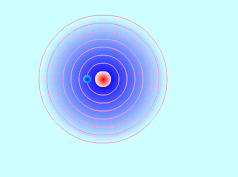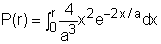Ch 8. Integration Techniques Multimedia Engineering Math Integration byParts Trig. IntegralsTrig. Subst. Fractions& Substitutions ApproximateIntegration
 Chapter 1. Limits 2. Derivatives I 3. Derivatives II 4. Mean Value 5. Curve Sketching 6. Integrals 7. Inverse Functions 8. Integration Tech. 9. Integrate App. 10. Parametric Eqs. 11. Polar Coord. 12. Series Appendix Basic Math Units Search eBooks Dynamics Fluids Math Mechanics Statics Thermodynamics Author(s): Hengzhong Wen Chean Chin Ngo Meirong Huang Kurt Gramoll ©Kurt GramollMATHEMATICS - CASE STUDY IntroductionElectron "Cloud" in an Atom A hydrogen atom is composed of one proton at the nucleus and one electron that moves around the nucleus. The electron does not move in a well-defined orbit but occupies a sphere shape "cloud" that surrounds the nucleus. At the lowest energy sate, the "cloud" can be assumed as a sphere centered at the nucleus. The way to describe the motion of the electron is to find the probability that the electron will be found within a certain area. What is known: The probability density function for the electron radial location is:       p(r) = 4/a3r2e-2x/a where a is a constant. Its integral gives the probability that the electron will be found within a certain area:where r is the radius of the sphere centered at the nucleus where the electron will be found and a is a constant. Questions Determine the probability that the electron can be within the spherical volume of radius of 3a. Approach Use integration by parts. Let:       u = x2       dv = e-2x/a dx